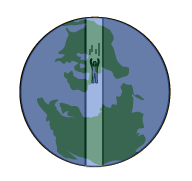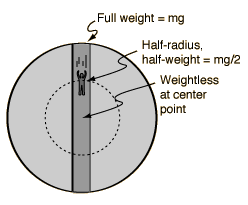# 从地球一端到另一端的地心之旅需要多久？\begin{equation*} g=\frac{GM_地}{R^2}=9.8\mathrm{m/s^2} \end{equation*}

\begin{equation*} g_{等效}=\frac{GM(r)}{r^2}=\frac{G}{r^2}\left (\rho\frac{4\pi}{3}r^3\right ) \end{equation*}

\begin{equation*} \rho=\frac{M_地}{\frac{4\pi}{3}R^3} \end{equation*}

\begin{equation*} g_{等效}=\frac{GM(r)}{r^2}=\frac{G}{r^2}\left (\rho\frac{4\pi}{3}r^3\right )=\frac{GM_地}{R^2}\frac{r}{R}=g\frac{r}{R} \end{equation*}\begin{equation*} F=-mg\frac{r}{R}=-kr \end{equation*}

\begin{equation*} \begin{split} T=&2\pi\sqrt{\frac{m}{k}}=2\pi\sqrt{\frac{m}{mg/R}}=2\pi\sqrt{\frac{R}{g}} \\ =&2\pi\sqrt{\frac{6.378\times 10^6}{9.8}}\mathrm s=5068\mathrm s=84.5 \mathrm {min} \end{split} \end{equation*}